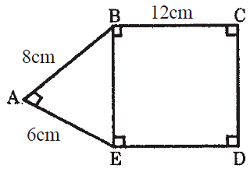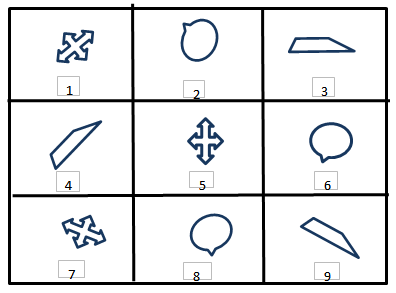# Indian Talent Olympiad - IMO PDF Sample Papers for Class 10

Class 10 sample paper & practice questions for International Maths Olympiad (IMO) level 1 are given below. Syllabus for level 1 is also mentioned for these exams. You can refer these sample paper & quiz for preparing for the exam.#### Resources:

##### Sample Questions from Olympiad Success:
 Q.1 Q.2 Q.3 Q.4 Q.5 Q.6 Q.7 Q.8 Q.9 Q.10
 Q.1 If A, B, D are 3 points in a ground. A is to the West of B, B is to the North of D, and D is to the South-East of A, what angles does BAD make? a) 180 b) 125 c) 90 d) 45
 Q.2 A dealer bought 80 cricket bats for ₹ 50 each. He sells 20 of them at a gain of 5%. What must be the gain percentage of the remaining bats, so as to get 10% gain on the whole? a) 3 2/11 % b) 12 1/2 % c) 11 2/3 % d) 4 2/3 %
 Q.3 Ankit and Anuj started simultaneously from X and Y respectively. Each person started towards the starting point of the other XY = 18 km. The speeds of Ankit and Anuj throughout their journeys were 5 kmph and 4 kmph respectively. On reaching X or Y, each turned back immediately to resume the journey to his starting point. Find the total distance travelled by Ankit before meeting Anuj for the second time. (in m) a) 30 b) 27 c) 24 d) 33
 Q.4 What will be the lateral surface area of a cylinder (in terms of radius r) whose height is twice of its radius? a) 2πr3 b) 4πr2 c) 6πr2 d) 8πr2
 Q.5 The following question is based on the following alphabet series. A B C D E F G H I J K L M N O P Q R S T U V W X Y Z What should come in place of ?Z, T, ?, H, B a) N b) L c) P d) None of these
 Q.6 The value of an old bike decreases every year at the rate of 4% over that of the previous year. If its value at the end of years is ₹ 13824, then find its present value. a) ₹ 15,625 b) ₹ 14,525 c) ₹ 16,625 d) ₹ 15,425
 Q.7 In figure AB = 8 cm, BC = 12 cm and AE = 6 cm. Find area of the rectangle BCDE.a) 120 cm² b) 190 cm² c) 220 cm² d) 150 cm²
 Q.8 Identify the identical figures from the following options given.a) (1, 5, 7), (3, 6, 8), (2, 4, 9) b) (1, 4, 7), (2, 6, 8), (3, 5, 9) c) (1, 5, 7), (2, 6, 8), (3, 4, 9) d) (5, 7, 9), (2, 6, 8), (1, 3, 4)
 Q.9 If diameter of cylinder is 8 cm and its height is 16 cm then volume of cylinder is: a) 804.352 cm3 b) 810.465 cm3 c) 750.352 cm3 d) 850.098 cm3
 Q.10 A sum of ₹ 24,000 is divided into two parts P1 and P2, such that the simple interest calculated on P1 for 3 year and on P2 for 4 years are equal. If the rates of interest on P1 and P2 are 4% and 5% respectively, then find the smaller of the parts P1 and P2. a) ₹ 10,000 b) ₹ 12,000 c) ₹ 8000 d) ₹ 9000Sample PDF of Indian Talent Olympiad - International Maths Olympiad (IMO) PDF Sample Papers for Class 10:

 Q.1 )d Q.2 )c Q.3 )a Q.4 )b Q.5 )a Q.6 )a Q.7 )a Q.8 )c Q.9 )a Q.10 )d

Q.1 : d | Q.2 : c | Q.3 : a | Q.4 : b | Q.5 : a | Q.6 : a | Q.7 : a | Q.8 : c | Q.9 : a | Q.10 : d